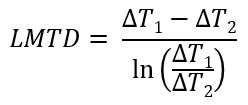# Understanding LMTD for heat exchanger design

## LMTD Definition - Log Mean Temperature Difference

Log Mean Temperature Difference (LMTD) is an indicator of the average temperature difference between the hot and cold fluids in a heat exchanger.

It is calculated using the following equation -ΔT1 → the temperature difference between hot and cold fluids at one end of the heat exchanger
ΔT2 → the temperature difference between hot and cold fluids at the other end of the heat exchanger

As can be seen from the equation, LMTD uses the log mean of the temperature differences instead of an arithmetic mean.

You can also use the calculator to quickly calculate the LMTD for a given exchanger.

## Using LMTD to calculate heat transfer

Following equation is used to calculate the overall heat transfer across a heat exchanger, using LMTD.

Q = U × Aoverall × LMTD ....... Equation (i)

Here,
Q is the overall heat transfer rate across a given heat exchanger (in Watts)
U is the overall heat transfer coefficient for that exchanger
Aoverall is the overall effective heat transfer area between the hot and cold sides of the exchanger
LMTD is the logarithmic mean of temperature differences as discussed above.

## How does LMTD impact heat exchanger design and selection?

Generally, you would use heat exchangers to heat up or cool down a 'process fluid'. This is done using a 'utility' which is either hotter or colder than the process fluid, depending on requirements.

Having a big temperature difference between hot and cold sides of an exchanger, certainly promotes better and faster heat transfer rate. Refer to the equation(i) from above.

So in case of a large LMTD, you would need lesser heat transfer area, implying that you can select a smaller heat exchanger with a simpler design, which is easier to maintain.

So in general you would be better off selecting a utility that is a lot hotter or lot cooler than your process fluid.

### Other precautions to be taken

If you consider other factors however, then the answer to this question may (or may not) change.

For example - if you consider temperature control for your process fluid, you would need a very finely tuned flow controller on the utility side. Because even a small variation in utility flow will be magnified into temperature changes on the process side.

## Calculating LMTD and understanding other nuances

Here is a quick tutorial for LMTD calculation.

Calculators -